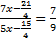## Ratio and Proportion For SBI PO : Set – 10

1) A certain amount to be distributed among A,B, and C in the ratio 2:3:4 respectively but was erroneously distributed in the ratio 7:2:5 respectively. As a result of this ,B got Rs. 40 less. What is the amount?

a) Rs.210

b) Rs.270

c) Rs.230

d) Rs.280

e) None of these

a)

Let the amount be X the distruibution For B is 3/9

The error amount calculated for B 2/14

By this we got Rs.40 Less

x/3-x/7=40

4x=40*210

x=210

2) A can contains a mixture of two liquids A and B is the ratio  7 : 5. When 9 litres of mixture are drawn off and the can is filled with B, the ratio of A and B becomes 7 : 9. How many litres of liquid A was contained by the can initially?

a) 10

b) 20

c) 21

d) 25

e) 22

c)

Quantity of A in mixture left = (7x-7/12*9)litres

= (7x-21/4) litres

Quantity of B in mixture left = (5x-5/12*9) litres

(5x-15/4) litres252x-189=140x=147

112x=336 x=3

Can Contains 21 litres of A

3) Mr. Xavier invested a certain amount in Debit and Equity funds in the ratio of 4 : 5 respectively. At the end of one year, he earned a total dividend of 30% on his investment. After one year he reinvested the amount including dividend in the ratio of 6 : 7 in Debt and Equity Funds. If the amount reinvested in Equity Funds was Rs. 94,500/-, what was the original amount invested in Equity Funds?

a) Rs. 75,000/-

b) Rs. 81,000/-

c) Rs. 60,000/-

d) Rs. 65,000/-

e) None of these

a) Amount invested in Debit funds = 4.

Amount invested in equity funds = 5.

After 1 yr Mr. Xaviers total amount =4x*130/100 +5x*130/100 => 11.7,

Amount invested in equity funds = 11.7 x 7/13

11.7 x 7/13= 94500

= 15000

The original amount invested in equity funds = 5 x 15000

= Rs. 75000

4) The ratio of the monthly salaries of A and B is in the ratio  15 : 16 and that of B and C is in the ratio 17 : 18. Find the monthly income of C if the total of their monthly salary is Rs 1,87,450.

a) Rs 66,240

b) Rs 72,100

c) Rs 62,200

d) Rs 65,800

e) Rs 60,300

a)

A: B = 15: 16

B: C = 17: 18

A: C = 255: 288

A: B: C = 255: 272: 288

C = (288/815)*187450

= 66240

5) The income of A, B, and C are in the ratio 3 : 4 : 7. If their incomes be changed such that the new income of A is 50% increased, 25% increased for B and 25% decrease for C. Find the ratio of their new incomes.

a) 18 : 40 : 23

b) 17 : 12 : 21

c) 18 : 20 : 21

d) 28 : 20 : 21

e) None of these

c)

A: B: C :: 3: 4: 7

New income ratio = 4.5: 5: 5.25

= 18: 20: 21

6) If Rs. 1540 be divided amongst A, B and C in such a way that the share of B is equal to of what A and C together receive. Then, B’s share will be:

a) Rs. 770

b) Rs. 420

c) Rs. 880

d) Rs. 1210

e) None of these

a)

if a get 100 and c get 200 then b get 300.

So the ratio is 1:3:2

B’s share= 1540*3/6 = 770

7) The ages of A, B and C are in the ratio 3:7:9. If the difference between the ages of B and C is 8 years, what is the sum of the ages of all the three together?

a) 40

b) 48

c) 64

d) 76

e) None of these

d)

8) A sum of money is divided among A, B, C and D in the ratio 3:7:9:13 respectively. If the share of B is Rs. 4,872, then what is the total amount of money of A and C together?

a) Rs. 9,784

b) Rs. 6,998

c) Rs. 8,352

d) Rs. 7, 456

e) None of these

c)

9) Rs. 1870 are divided into three parts in such a way that half of the first part, one-third of the second part and one-sixth of the third part are equal. The third part is :

a) Rs. 510

b) Rs. 680

c) Rs. 850

d) Rs. 1020

e) none of these

d)

10) In a bag, there are three types of coins — 1-rupee, 50 paise and 25-paise in the ratio of 3 : 8 : 20. Their total value is Rs. 372. The total number of coins is

a) 1200

b) 961

c) 744

d) 612

e) None of these